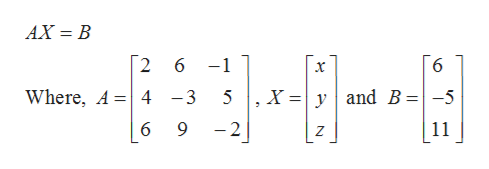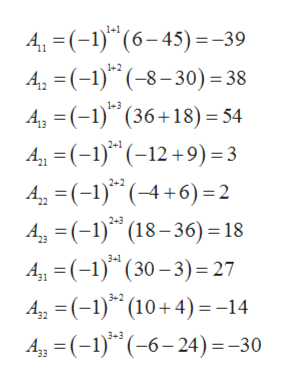# Solve for (x,y,z)for the following three equations using direct matrix inversion method (1). 2x+6y-z=6(2). 4x-3y+5z=-5(3). 6x+9y-2z=11

Question
6 views

Solve for (x,y,z)for the following three equations using direct matrix inversion method

(1). 2x+6y-z=6

(2). 4x-3y+5z=-5

(3). 6x+9y-2z=11

check_circle

Step 1

The given system of equation can be written in matrix form as,help_outlineImage TranscriptioncloseAX = B 2 6 6 -1 x Where, A 4 yand B -5 9 11 -2 Z fullscreen
Step 2

Now

Step 3

Now we find the cofactors of ...help_outlineImage Transcriptionclose4 (-1) (6-45) = -39 A ()(8-30) = 38 Ag (1) (36+18) 54 A21 (-1) (-12+9) =3 4(-)(4+6)2 42(-1) (18-36) 18 4, (-) (30-3) 27 42 (-1) (10+4)= -14 A(1) 6-24)=-30 1+1 1+2 1+3 2+2 2+3 3+1 3+2 3+3 fullscreen

### Want to see the full answer?

See Solution

#### Want to see this answer and more?

Solutions are written by subject experts who are available 24/7. Questions are typically answered within 1 hour.*

See Solution
*Response times may vary by subject and question.
Tagged in

### Algebra